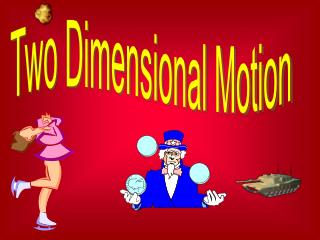Download PresentationFour Major Types of Two Dimensional Motion

Four Major Types of Two Dimensional Motion - PowerPoint PPT Presentation

Four Major Types of Two Dimensional Motion. 1. Projectile Motion 2. Circular Motion 3. Rotational Motion 4. Periodic Motion. Projectile motion problems are best solved by treating horizontal and vertical motion separately. * IMPORTANT * Gravity only affects vertical motion.I am the owner, or an agent authorized to act on behalf of the owner, of the copyrighted work described.
Download PresentationFour Major Types of Two Dimensional Motion

Download Policy: Content on the Website is provided to you AS IS for your information and personal use and may not be sold / licensed / shared on other websites without getting consent from its author.While downloading, if for some reason you are not able to download a presentation, the publisher may have deleted the file from their server.

- - - - - - - - - - - - - - - - - - - - - - - - - - E N D - - - - - - - - - - - - - - - - - - - - - - - - - -
Presentation Transcript

1. Four Major Types of Two Dimensional Motion 1. Projectile Motion 2. Circular Motion 3. Rotational Motion 4. Periodic Motion

2. Projectile motion problems are best solved by treating horizontal and vertical motion separately. *IMPORTANT* Gravity only affects vertical motion. There are two general types of projectile motion situations. 1. object launched horizontally 2. object launched at an angle

3. Object Launched Horizontally vx = initial horizontal velocity t = total time in the air “Hang Time” Dy = initial height above ground Dx = horizontal range IMPORTANT FACTS There is no horizontal acceleration. There is no initial vertical velocity. The horizontal velocity is constant. Time is the same for both vertical and horizontal. vertical horizontal Dx = Vxt D = 0.5gt2

4. Object Launched at an Angle V = initial velocity q = launch angle Dy = maximum height t = Hang Time = total time in air Dx = horizontal range IMPORTANT FACTS The horizontal velocity is constant. It rises and falls in equal time intervals. It reaches maximum height in half the total time. Gravity only effects the vertical motion. horizontal Vx = V cosq Dx = vxt vertical Vy = V sinq Dy = 0.5(0.5)Vyt t = -2Vy/g

6. object moves in circular path about an external point (“revolves”)

7. According to Newton’s First Law of Motion, objects move in a straight line unless a force makes them turn. An external force is necessary to make an object follow a circular path. This force is called a CENTRIPETAL (“center seeking”) FORCE. Since every unbalanced force causes an object to accelerate in the direction of that force (Newton’s Second Law), a centripetal force causes a CENTRIPETAL ACCELERATION. This acceleration results from a change in direction, and does not imply a change in speed, although speed may also change.

8. Centripetal force and acceleration may be caused by: • gravity - planets orbiting the sun • friction - car rounding a curve • a rope or cord - swinging a mass on a string In all cases, a mass m moves in a circular path of radius r with a linear speed v. The time to make one complete revolution is known as the period, T. The speed v is the circumference divided by the period. v = 2pr/T

9. The formula for centripetal acceleration is: ac= v2/r and centripetal force is: Fc=mac=mv2/r m = mass in kg v = linear velocity in m/s Fc = centripetal force in N r = radius of curvature in m ac = centripetal acceleration in m/s2

10. G Forces are sometimes confused with pulling G’s. G’s are multiples of the acceleration of gravity. Puling 4 G’s really means having a centripetal acceleration of 4 x 9.8 = 39.2 m/s/s